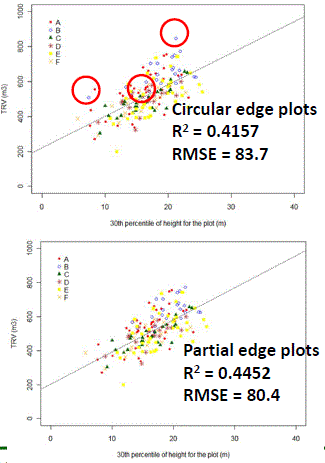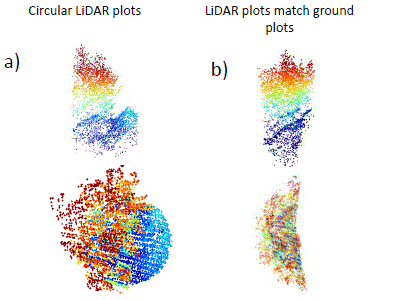+64 7 350 3209 +61 2 8011 3645 info@interpine.co.nz
Select Page

Interpine have been doing several research projects looking at the potential of using LiDAR as an auxiliary variable in regression sampling. The results are looking very promising and we have a range of production field trials underway to move the application of LiDAR into the practical forestry applications.

During this work we ran into the problem of how to deal with forest edge plots that have been measured using the mirage plot technique. Edge plots occur at the following stand boundaries: between two stands, road edge and external edges.

The following is an example of the impact of edge plots on the relationship between total recoverable and 30th percentile height metric at a plot level.   In the first graph the circuled plots are edge plots within the sample population, showing that several of the outliers are actually these edge plots.   The second graph is the slicing of these edge plots for recalculation of LiDAR metrics before placing back into the model, showing a direct improvement in the model fit and removal of these outliers.

Of course to do this correctly it is important that ground plots have been geo-located accuracy using Survey Grade GPS/GNSS receivers, as these forest edges are often of a very hetrogenous forest type. (see more information about accuracy requirements of fixing LiDAR ground controls)We welcome readers comments on this, as we are continuing for work on operational solutions to dealing with forest edges as it relates to LiDAR ground control surveys.

Example of “carving out LiDAR for forest edges”